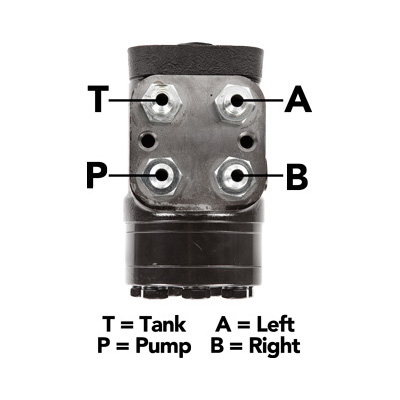# 100ml Orbital Steering Valve

\$274.99
(No reviews yet)
SKU:
SKU-2570-None
UPC:
305484204808
Availability:
48 - 72 Hr Processing Time
Steering orbital valve. 100ml. Comes with 4 x #6 Fittings.
This Valve is

The displacement of the valve divided into the steering cylinder volume will determine the required turns of the steering wheel to turn the steering axle full lock to lock.How to Calculate Full Hydraulic Steering

The basic formula for figuring out how many turns lock to lock of your steering system is to take the volume of the cylinder and divide it by the output displacement of the steering valve.

Volume of cylinder / cu in of orbital = turns lock to lock

example 25.82/7.6=3.39 turns lock to lock

Cylinder volume formula = piston area x stroke
(note you must subtract the area or volume that the rod displaces when figuring the volume of a cylinder on the rod side of the cylinder)

area of a circle formula = 3.14(pi) x radius squared

Example - a 1.75 bore cylinder has 1.75 diameter, which has a radius of .75 (diameter divided in half = radius). 3.14 x .75 x .75 = a piston area of 1.766 you then multiply that by your stroke to get displacement area or volume of your cylinder. 8 x 1.766 = 14.13 volume area.

You also can take your piston area and multiply that by your pump pressure to figure the force that the cylinder is able to produce.

80ml Orbital Steering Valve